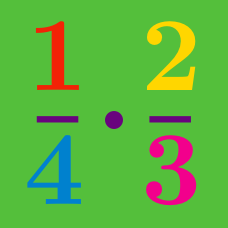Basic Mathematics

# Componendo and Dividendo

If $$\displaystyle \frac{a}{b} = \frac{3}{14},$$ what is $$\displaystyle \frac{b-a}{b+a}?$$

If $$\displaystyle \frac{x^2+8x-9}{x^2-8x-9} = \frac{x+9}{x-9},$$ what is $$x?$$

If $$\frac{a-b}{a+b} = \frac{ 3} { 8 }$$, then find the value of

$\frac{ 5a + 3b } { 5a - 3b }.$

If $$\displaystyle \frac{\sqrt{7}+x}{\sqrt{7}-x} = 7,$$ what is $$x?$$

If $$\displaystyle \frac{20+x}{20-x} = 4,$$ what is $$x?$$

×